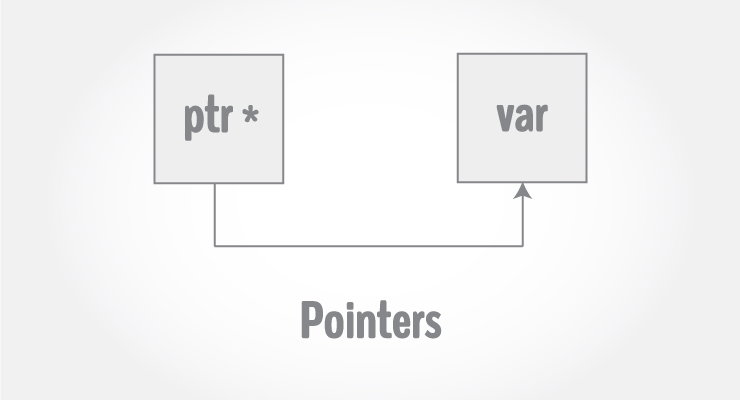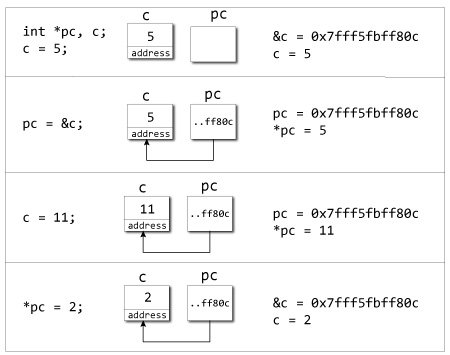# C++ Pointers

In this article, you'll learn everything about pointers. You'll learn how values are stored in the computer and how to access them using pointers.Pointers are powerful features of C++ that differentiates it from other programming languages like Java and Python.

Pointers are used in C++ program to access the memory and manipulate the address.

To understand pointers, you should first know how data is stored on the computer.

Each variable you create in your program is assigned a location in the computer's memory. The value the variable stores is actually stored in the location assigned.

To know where the data is stored, C++ has an & operator. The & (reference) operator gives you the address occupied by a variable.

If `var` is a variable then, `&var` gives the address of that variable.

## Example 1: Address in C++

``````#include <iostream>
using namespace std;

int main()
{
int var1 = 3;
int var2 = 24;
int var3 = 17;
cout << &var1 << endl;
cout << &var2 << endl;
cout << &var3 << endl;
}``````

Output

```0x7fff5fbff8ac
0x7fff5fbff8a8
0x7fff5fbff8a4
```

Note: You may not get the same result on your system.

The 0x in the beginning represents the address is in hexadecimal form.

Notice that first address differs from second by 4-bytes and second address differs from third by 4-bytes.

This is because the size of integer (variable of type `int`) is 4 bytes in 64-bit system.

## Pointers Variables

C++ gives you the power to manipulate the data in the computer's memory directly. You can assign and de-assign any space in the memory as you wish. This is done using Pointer variables.

Pointers variables are variables that points to a specific address in the memory pointed by another variable.

### How to declare a pointer?

```int *p;
OR,
int* p;
```

The statement above defines a pointer variable p. It holds the memory address

The asterisk is a dereference operator which means pointer to.

Here, pointer p is a pointer to `int`, i.e., it is pointing to an integer value in the memory address.

### Reference operator (&) and Deference operator (*)

Reference operator (&) as discussed above gives the address of a variable.

To get the value stored in the memory address, we use the dereference operator (*).

For example: If a number variable is stored in the memory address 0x123, and it contains a value 5.

The reference (&) operator gives the value 0x123, while the dereference (*) operator gives the value 5.

Note: The (*) sign used in the declaration of C++ pointer is not the dereference pointer. It is just a similar notation that creates a pointer.

## Example 2: C++ Pointers

C++ Program to demonstrate the working of pointer.

``````#include <iostream>
using namespace std;
int main() {
int *pc, c;

c = 5;
cout << "Address of c (&c): " << &c << endl;
cout << "Value of c (c): " << c << endl << endl;

pc = &c;    // Pointer pc holds the memory address of variable c
cout << "Address that pointer pc holds (pc): "<< pc << endl;
cout << "Content of the address pointer pc holds (*pc): " << *pc << endl << endl;

c = 11;    // The content inside memory address &c is changed from 5 to 11.
cout << "Address pointer pc holds (pc): " << pc << endl;
cout << "Content of the address pointer pc holds (*pc): " << *pc << endl << endl;

*pc = 2;
cout << "Address of c (&c): " << &c << endl;
cout << "Value of c (c): " << c << endl << endl;

return 0;
}``````

Output

```Address of c (&c): 0x7fff5fbff80c
Value of c (c): 5

Address that pointer pc holds (pc): 0x7fff5fbff80c
Content of the address pointer pc holds (*pc): 5

Address pointer pc holds (pc): 0x7fff5fbff80c
Content of the address pointer pc holds (*pc): 11

Value of c (c): 2```Explanation of program

• When `c = 5;` the value 5 is stored in the address of variable c - 0x7fff5fbff8c.
• When `pc = &c;` the pointer pc holds the address of c - 0x7fff5fbff8c, and the expression (dereference operator) `*pc` outputs the value stored in that address, 5.
• When `c = 11;` since the address pointer pc holds is the same as c - 0x7fff5fbff8c, change in the value of c is also reflected when the expression `*pc` is executed, which now outputs 11.
• When `*pc = 2;` it changes the content of the address stored by pc - 0x7fff5fbff8c. This is changed from 11 to 2. So, when we print the value of c, the value is 2 as well.

## Common mistakes when working with pointers

Suppose, you want pointer pc to point to the address of c. Then,

```int c, *pc;
pc=c;  /* Wrong! pc is address whereas, c is not an address. */
*pc=&c; /* Wrong! *pc is the value pointed by address whereas, %amp;c is an address. */
pc=&c; /* Correct! pc is an address and, %amp;pc is also an address. */
*pc=c; /* Correct! *pc is the value pointed by address and, c is also a value. */```

In both cases, pointer pc is not pointing to the address of c.

You should also check out these pointer related tutorials: